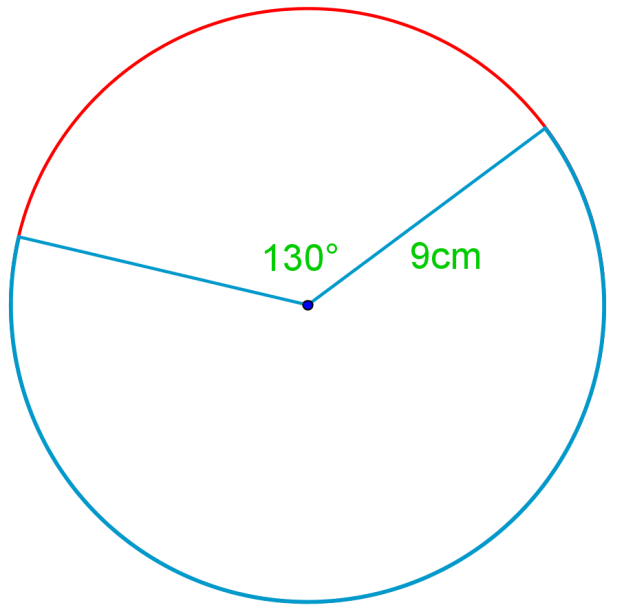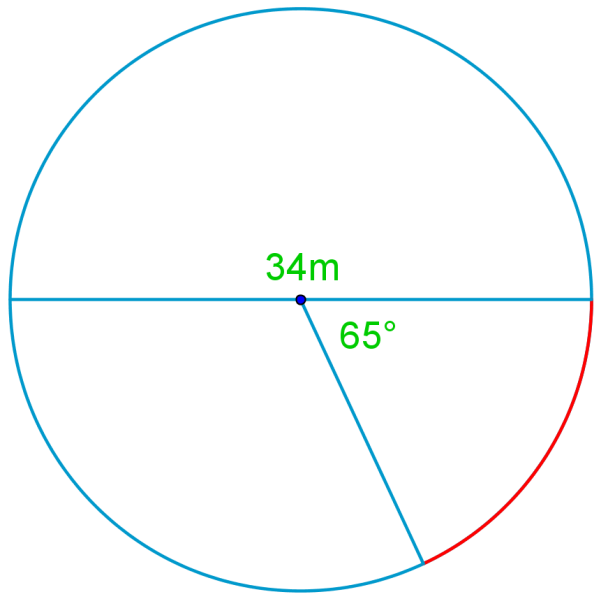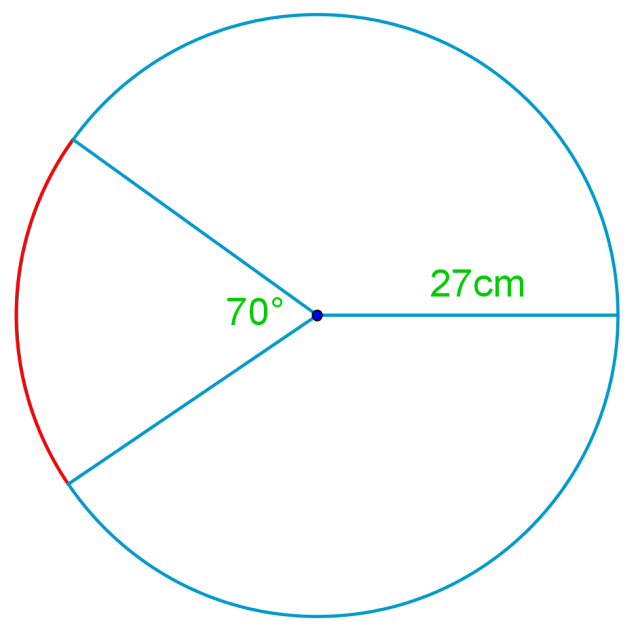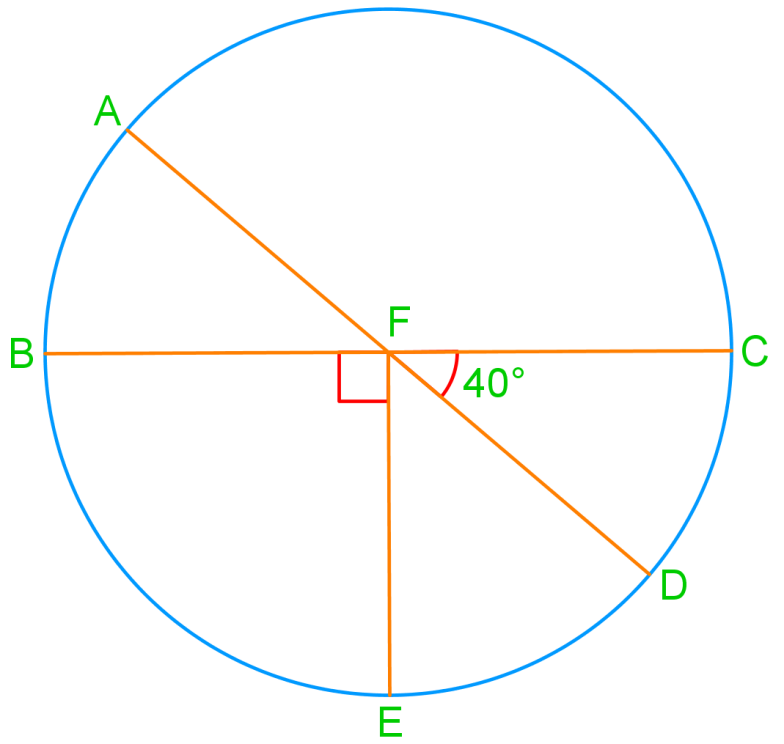# Arcs of a circle

### Arcs of a circle

#### Lessons

• Introduction
a)
Terms related to circles
• Radius
• Diameter
• Circumference
• Central angle
• minor arc
• major arc
• Inscribed angle
• Chord
• perpendicular bisector
• Tangent
• point of tangency

b)
How to find the length of an arc?
• by Central angle
• by Proportion
• by Using formula a = Θr

• 1.
Find the length of the arc in red.
a)b)c)• 2.
In the following circle, AD is diameter and BC $\bot$ EF, and the radius is 7. Find the measures of:a)
$arc AB$

b)
$arc ABE$

c)
$arc AEC$

d)
$\angle BFD$

• 3.
On circle D, arcAB= 105°, arcBC= 140° and arcABC= 245°. Point E is on the circle D too and arcCE= 145°. Explain what E must be on ArcAB.